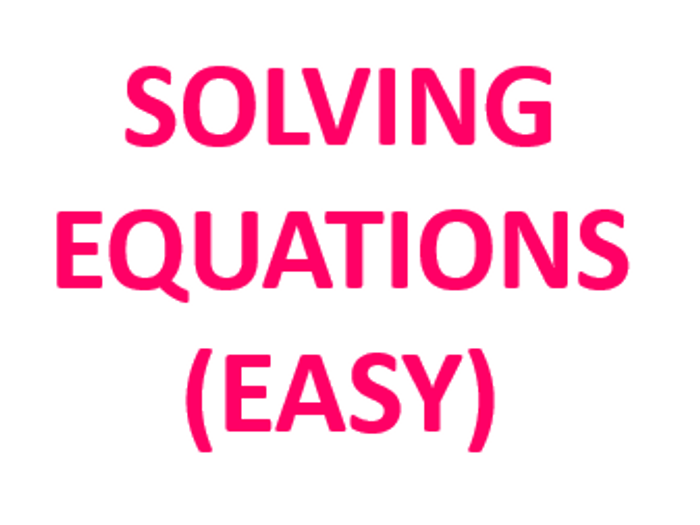This is a set of solving one-step and two step equations worksheets that can be used for classroom practise, as revision worksheets or as homework sheets. All worksheets come with answers.

It includes:

2. Solving 1-step equations - multiplication + answers

3. Solving 1- step equations - division + answers

4. Solving 2- step equations + answers

5. Solving 2- step equations harder questions+ answers

6. Solving equations mixed + answers
\$2.57
Save for later

Info

Created: Feb 12, 2015

Updated: Mar 10, 2019

Worksheet

pdf, 359 KB

Solving--Equations

Report a problem